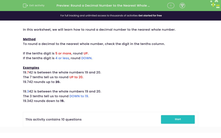# Round a Decimal Number to the Nearest Whole Number

In this worksheet, students will round decimal numbers to the nearest whole number.Key stage:  KS 3

Curriculum topic:   Number

Curriculum subtopic:   Round/Approximate Numbers Appropriately

Popular topics:   Numbers worksheets

Difficulty level:#### Worksheet Overview

In this activity, we will learn how to round a decimal number to the nearest whole number.

Method

To round a decimal to the nearest whole number, check the digit in the tenths column.

If the tenths digit is 5 or more, round up.

If the tenths digit is 4 or less, round down.

Examples

19.742 is between the whole numbers 19 and 20.

The 7 tenths tells us to round up to 20.

19.742 rounds up to 20.

19.342 is between the whole numbers 19 and 20.

The 3 tenths tells us to round down to 19.

19.342 rounds down to 19.

Are you ready to start rounding some decimals?### What is EdPlace?

We're your National Curriculum aligned online education content provider helping each child succeed in English, maths and science from year 1 to GCSE. With an EdPlace account you’ll be able to track and measure progress, helping each child achieve their best. We build confidence and attainment by personalising each child’s learning at a level that suits them.

Get started